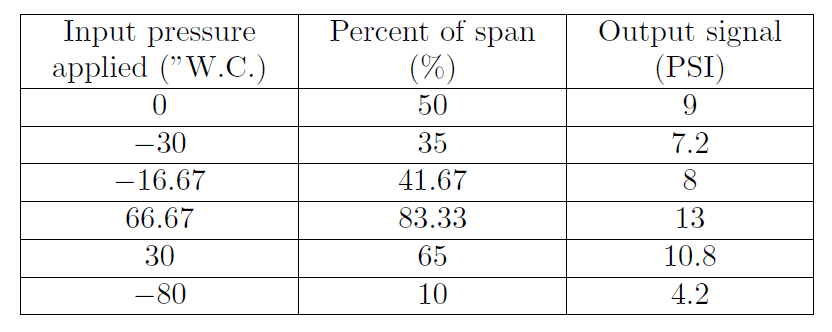# Calculate the Transmitter Values

A pneumatic differential pressure transmitter has a calibrated range of −100 to +100 inches of water column (” W.C.), and its output signal range is 3 to 15 PSI.

Complete the following table of values for this transmitter, assuming perfect calibration (no error).

## TransmitterDevelop a linear equation in the form of y = mx + b that directly relates input pressure (x) to output pressure (y).

Demonstrate how to estimate numerical answers for this problem without using a calculator.Explain the formula used for the above calculations.